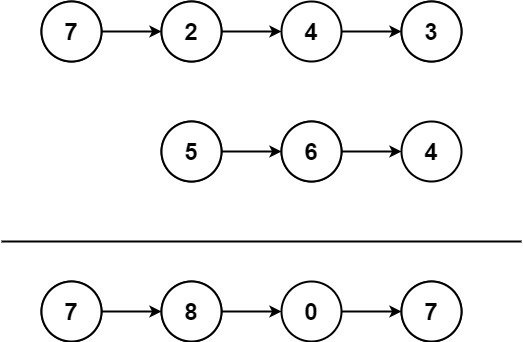## Algorithm

Problem Name: 445. Add Two Numbers II

You are given two non-empty linked lists representing two non-negative integers. The most significant digit comes first and each of their nodes contains a single digit. Add the two numbers and return the sum as a linked list.

You may assume the two numbers do not contain any leading zero, except the number 0 itself.

Example 1:```Input: l1 = [7,2,4,3], l2 = [5,6,4]
Output: [7,8,0,7]
```

Example 2:

```Input: l1 = [2,4,3], l2 = [5,6,4]
Output: [8,0,7]
```

Example 3:

```Input: l1 = , l2 = 
Output: 
```

Constraints:

• The number of nodes in each linked list is in the range `[1, 100]`.
• `0 <= Node.val <= 9`
• It is guaranteed that the list represents a number that does not have leading zeros.

## Code Examples

### #1 Code Example with Java Programming

```Code - Java Programming```

``````
class Solution {
public ListNode addTwoNumbers(ListNode l1, ListNode l2) {
ListNode revL1 = reverse(l1);
ListNode revL2 = reverse(l2);
ListNode dummy = new ListNode(0);
ListNode curr = dummy;
int carry = 0;
while (revL1 != null || revL2 != null || carry != 0) {
if (revL1 != null && revL2 != null) {
carry += revL1.val + revL2.val;
revL1 = revL1.next;
revL2 = revL2.next;
} else if (revL1 != null && revL2 == null) {
carry += revL1.val;
revL1 = revL1.next;
} else if (revL1 == null && revL2 != null) {
carry += revL2.val;
revL2 = revL2.next;
}
curr.next = new ListNode(carry % 10);
carry /= 10;
curr = curr.next;
}
return reverse(dummy.next);
}

private ListNode reverse(ListNode root) {
ListNode curr = root;
ListNode prev = null;
while (curr != null) {
ListNode next = curr.next;
curr.next = prev;
prev = curr;
curr = next;
}
return prev;
}
}
``````
Copy The Code &

Input

cmd
l1 = [7,2,4,3], l2 = [5,6,4]

Output

cmd
[7,8,0,7]

### #2 Code Example with Javascript Programming

```Code - Javascript Programming```

``````
let l1 = r1, l2 = r2, inc = 0
let dummy = new ListNode()
let pre = dummy
while(l1 || l2) {
let val = inc
if(l1) {
val += l1.val
l1 = l1.next
}
if(l2) {
val += l2.val
l2 = l2.next
}
if(val > 9) inc = 1
else inc = 0
const cur = new ListNode(val % 10)
pre.next = cur
pre = cur
}
if (inc) {
pre.next = new ListNode(1)
}
return reverse(dummy.next)
};

const dummy = new ListNode()
let len = 0, cur = head
while(cur) {
len++
cur = cur.next
}
let p = dummy, tail = head, tmp = null
for(let i = 0; i < len - 1; i++) {
tmp = p.next
p.next = tail.next
tail.next = tail.next.next
p.next.next = tmp
}
return dummy.next
}
``````
Copy The Code &

Input

cmd
l1 = [7,2,4,3], l2 = [5,6,4]

Output

cmd
[7,8,0,7]

### #3 Code Example with Python Programming

```Code - Python Programming```

``````
class Solution:
def addTwoNumbers(self, l1: ListNode, l2: ListNode) -> ListNode:
s1, s2, s3 = [], [], []
p1, p2 = l1, l2
while p1:
s1.append(p1.val)
p1 = p1.next
while p2:
s2.append(p2.val)
p2 = p2.next
if len(s1) < len(s2):
s1, s2 = s2, s1
l1, l2 = l2, l1
residual = 0
while len(s1) > 0:
temp = s1.pop() + residual
if len(s2) > 0:
temp += s2.pop()
s3.append(temp % 10)
residual = temp // 10
while len(s3) > 0:
p.val = s3.pop()
p = p.next
``````
Copy The Code &

Input

cmd
l1 = [2,4,3], l2 = [5,6,4]

Output

cmd
[8,0,7]

### #4 Code Example with C# Programming

```Code - C# Programming```

``````
using System.Collections.Generic;

namespace LeetCode
{
{
public ListNode AddTwoNumbers(ListNode l1, ListNode l2)
{
var stack1 = new Stack();
var stack2 = new Stack();
while (l1 != null)
{
stack1.Push(l1.val);
l1 = l1.next;
}
while (l2 != null)
{
stack2.Push(l2.val);
l2 = l2.next;
}

var sum = 0;
ListNode current = null;
while (stack1.Count > 0 || stack2.Count > 0 || sum > 0)
{
if (stack1.Count > 0)
sum += stack1.Pop();
if (stack2.Count > 0)
sum += stack2.Pop();

var newNode = new ListNode(sum % 10, current);
current = newNode;
sum /= 10;
}

return current;
}
}
}
``````
Copy The Code &

Input

cmd
l1 = [2,4,3], l2 = [5,6,4]

Output

cmd
[8,0,7]